# Examples in R

## Using Mean Differences

If we were to generate some random data from a normal distribution with the following code,

GroupA <- rnorm(20)
GroupB <- rnorm(20)

RandomData <- data.frame(GroupA, GroupB)

and compare the means of these two “groups” using a t-test,

testresults <- t.test(GroupA, GroupB, data = RandomData, paired = FALSE)

we would likely see some differences, given that we have such a small sample in each group.

summary(testresults)

We can see our P-value for the statistical test along with the computed 95% interval with the command above (which is given to us by default by the program). Thus, effect sizes that range from the lower bound of this interval to the upper bound are compatible with the test model at this consonance level.

However, as stated before, a 95% interval is only an artifact of the commonly used 5% alpha level for hypothesis testing and is nowhere near as informative as a function.

If we were to take the information from this data and calculate a P-value function where every single consonance interval and its corresponding P-value were plotted, we would be able to see the full range of effect sizes compatible with the test model at various levels.

It is relatively easy to produce such a function using the concurve package in R.

Install the package directly from CRAN

install.packages("concurve")

or get a more slightly up-to-date version via GitHub.

library(devtools)
install_github("zadchow/concurve")

We’ll use the same data from above to calculate a P-value function and since we are focusing on mean differences using a t-test, we will use the curve_meta() function to calculate our consonance intervals and store them in a dataframe.

library(concurve)
intervalsdf <- curve_mean(GroupA, GroupB,
data = RandomData, method = "default"
)

Now thousands of consonance intervals at various levels have been stored in the dataframe “intervalsdf.” We can preview some of the entries via the tibble package.

tibble::tibble(intervalsdf)

There’s a quick look at the first few entries of our dataframe.

We can plot this data using the ggconcurve() function.

pfunction <- ggconcurve(type = "consonance", intervalsdf)
pfunction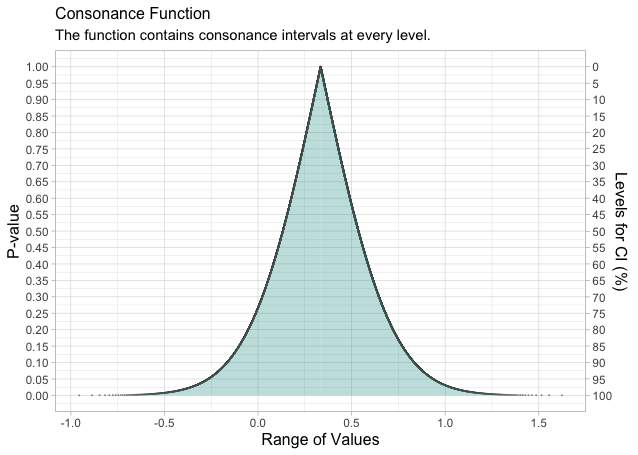Now we can see every consonance interval and its corresponding P-value and consonance level plotted. As stated before, a single 95% consonance interval is simply a slice through this function, which provides far more information as to what is compatible with the test model and its assumptions.

Furthermore, we can also produce a “surprisal” function by plotting every consonance interval and its corresponding S-value using the ggconcurve() function with type being set as “surprisal”.

sfunction <- ggconcurve(type = "surprisal", intervalsdf)
sfunction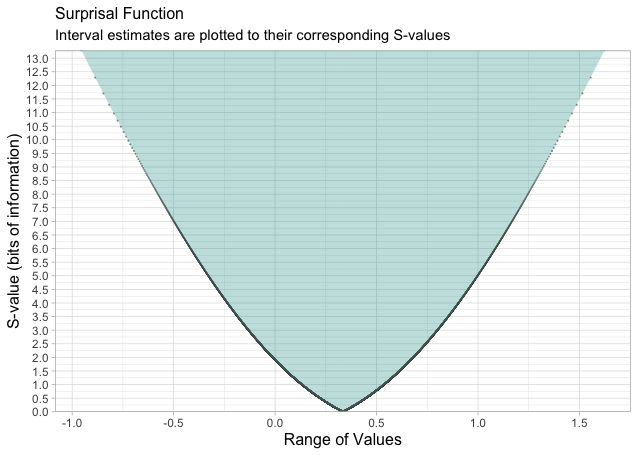The graph from the code above provides us with consonance levels and the maximum amount of information against the effect sizes contained in the consonance interval.

## Simple Linear Models

We can also try this with other simple linear models.

Let’s simulate more normal data and fit a simple linear regression to it using ordinary least squares regression with the base R lm() function.

GroupA2 <- rnorm(500)
GroupB2 <- rnorm(500)

RandomData2 <- data.frame(GroupA2, GroupB2)

model <- lm(GroupA2 ~ GroupB2, data = RandomData2)

summary(model)

We can see some of the basic statistics of our model including the 95% interval for our predictor (GroupB). Perhaps we want more information. Well we can do that! Using the curve_gen(), we can calculate several consonance intervals for the regression coefficient and then plot the consonance and surprisal functions.

randomframe <- curve_gen(model, "GroupB2")
tibble::tibble(randomframe)

Now that we have our data frame, we can graph our function.

ggconcurve(type = "consonance", randomframe)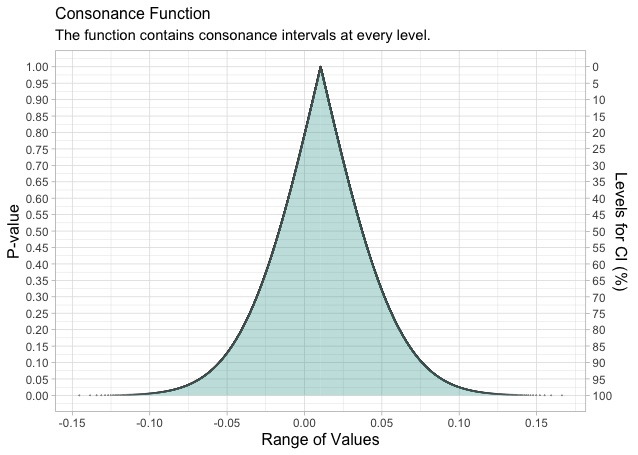s <- ggconcurve(type = "surprisal", randomframe)
s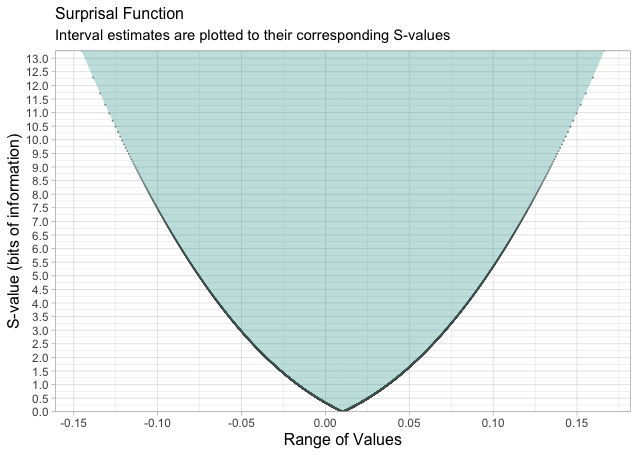We can also compare these functions to likelihood functions (also called support intervals), and we’ll see that we get very similar results. We’ll do this using the ProfileLikelihood package.

xx <- profilelike.lm(
formula = GroupA2 ~ 1, data = RandomData2,
profile.theta = "GroupB2",
lo.theta = -0.3, hi.theta = 0.3, length = 500
)

Now we plot our likelihood function and we can see what the maximum likelihood estimation is. Notice that it’s practically similar to the interval in the S-value function with 0 bits of information against it and and the consonance interval in the P-value function with a P-value of 1.

profilelike.plot(
theta = xx$theta, profile.lik.norm = xx$profile.lik.norm, round = 3
)
title(main = "Likelihood Function")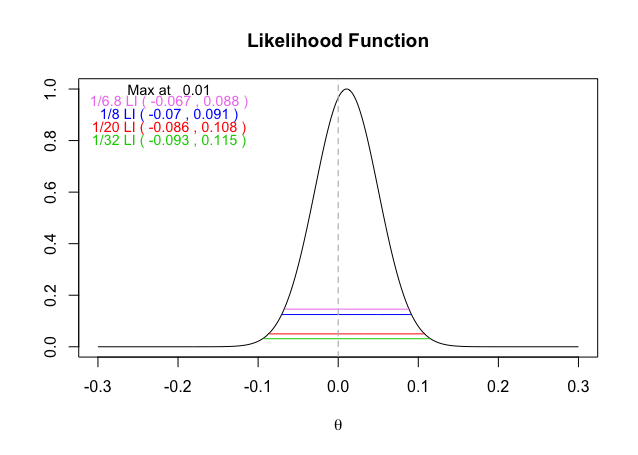We’ve used a relatively easy example for this blog post, but the concurve package is also able to calculate consonance functions for multiple regressions, logistic regressions, ANOVAs, and meta-analyses (that have been produced by the metafor package).

## Using Meta-Analysis Data

Here we present another quick example with a meta-analysis of simulated data.

First, we generate random data for two groups in two hypothetical studies

GroupAData <- runif(20, min = 0, max = 100)
GroupAMean <- round(mean(GroupAData), digits = 2)
GroupASD <- round(sd(GroupAData), digits = 2)

GroupBData <- runif(20, min = 0, max = 100)
GroupBMean <- round(mean(GroupBData), digits = 2)
GroupBSD <- round(sd(GroupBData), digits = 2)

GroupCData <- runif(20, min = 0, max = 100)
GroupCMean <- round(mean(GroupCData), digits = 2)
GroupCSD <- round(sd(GroupCData), digits = 2)

GroupDData <- runif(20, min = 0, max = 100)
GroupDMean <- round(mean(GroupDData), digits = 2)
GroupDSD <- round(sd(GroupDData), digits = 2)

We can then quickly combine the data in a dataframe.

StudyName <- c("Study1", "Study2")
MeanTreatment <- c(GroupAMean, GroupCMean)
MeanControl <- c(GroupBMean, GroupDMean)
SDTreatment <- c(GroupASD, GroupCSD)
SDControl <- c(GroupBSD, GroupDSD)
NTreatment <- c(20, 20)
NControl <- c(20, 20)

StudyName, MeanTreatment, MeanControl,
SDTreatment, SDControl,
NTreatment, NControl
)

Then, we’ll use metafor to calculate the standardized mean difference.

dat <- escalc(
measure = "SMD",
m1i = MeanTreatment, sd1i = SDTreatment, n1i = NTreatment,
m2i = MeanControl, sd2i = SDControl, n2i = NControl,
)

Next, we’ll pool the data using a fixed-effects common-effects model

res <- rma(yi, vi,
data = dat, slab = paste(StudyName, sep = ", "),
method = "FE", digits = 2
)

Let’s look at our output.

res
Fixed-Effects Model (k = 2)

Test for Heterogeneity:
Q(df = 1) = 0.61, p-val = 0.44

Model Results:

estimate    se  zval  pval  ci.lb  ci.ub
0.16  0.22  0.73  0.47  -0.28   0.60

---
Signif. codes:  0 ‘***’ 0.001 ‘**’ 0.01 ‘*’ 0.05 ‘.’ 0.1 ‘ ’ 1 

Take a look at the pooled summary effect and its interval. Keep it in mind as we move onto constructing a consonance function.

We can now take the object produced by the meta-analysis and calculate a P-value and S-value function with it to see the full spectrum of effect sizes compatible with the test model at every level. We’ll use the curve_meta() function to do this.

metaf <- curve_meta(res)
tibble::tibble(metaf)

Now that we have our dataframe with every computed interval, we can plot the functions.

ggconcurve(type = "consonance", metaf)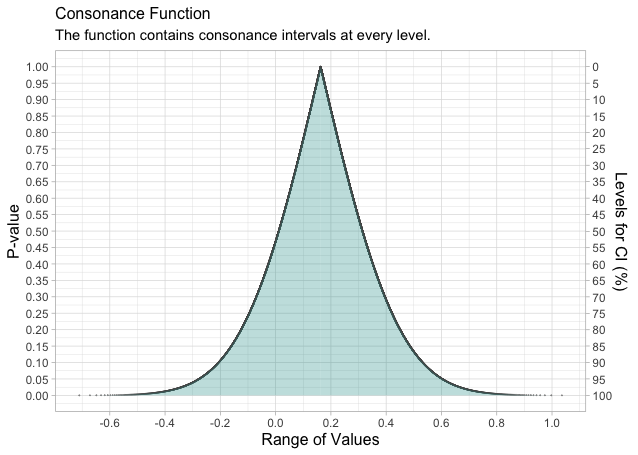And our S-value function

ggconcurve(type = "surprisal", metaf)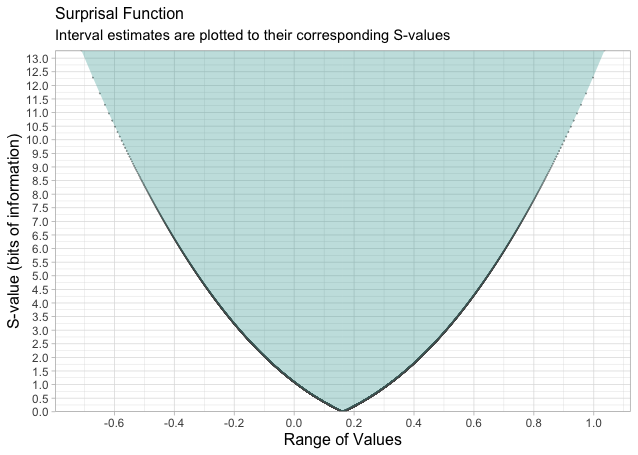Compare the span of these functions and the information they provide to the consonance interval provided by the forest plot. We are now no longer limited to interpreting an arbitrarily chosen interval by mindless analytic decisions often built into statistical packages by default.